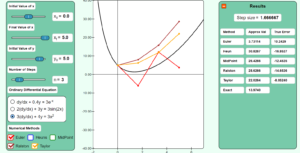# Chapter 08 Runge-Kutta 2nd Order Method Simulation

Description:  This simulation shows how Runge-Kutta 2nd order method works to solve ordinary differential equations of the form dy/dx=f(x,y), y(x0)=y0.Keywords: Ordinary differential equation, numerical methods, Runge-Kutta 2nd order method, Taylor series, Euler’s method.

Learning Objectives:  After successful completion of running the simulation with proper questions asked by the instructor, the student would be able to 1) note how the Runge-Kutta 2nd order methods of solving ordinary differential equations work 2) study the effect of step size on the convergence of the methods 3) compare results with exact, Euler’s method, and directly using the first three terms of the Taylor series.

Full Resources:  The full resources for the topic include textbook content,  PowerPoint presentations, multiple-choice tests, audiovisual lectures, and application examples.